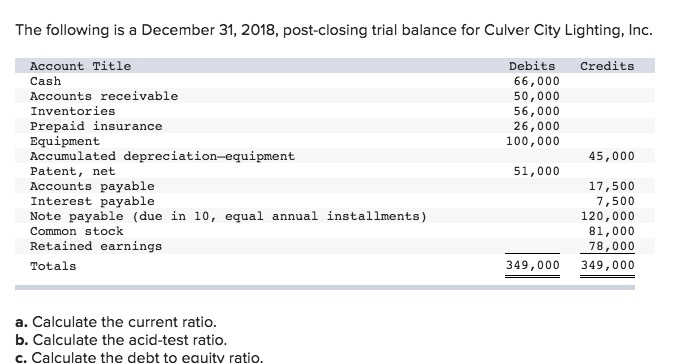# Question & Answer: The following is a December 31, 2018, post-closing trial balance for Culver City Lighting, Inc. a. Calculate the current ratio. b…..The following is a December 31, 2018, post-closing trial balance for Culver City Lighting, Inc. a. Calculate the current ratio. b. Calculate the acid-test ratio. c. Calculate the debt to equity ratio.

Don't use plagiarized sources. Get Your Custom Essay on
Question & Answer: The following is a December 31, 2018, post-closing trial balance for Culver City Lighting, Inc. a. Calculate the current ratio. b…..
GET AN ESSAY WRITTEN FOR YOU FROM AS LOW AS \$13/PAGE

Total current assets=(Cash+Accounts receivable+Inventories+PRepaid insurance)

=(\$66000+\$50000+\$56000+26000)=\$198000

Total current liabilities=(Accounts payable+Interest payable+Current portion of notes payable_)(Current portion of notes payable is the balance in next 12 months=(\$120,000/10)=\$12000)

=(\$17500+7500+12000)=\$37000

Hence

1.Current ratio=Current assets/Current liabilities

=(\$198000/\$37000)=5.35(Approx)

2.Acid test ratio=(Current assets-inventory-prepaid expenses)/Current liabilities

=(\$198000-56000-26000)/37000=3.14(Approx).

3.

Total debt=(Interest payable+Accounts payable+Notes payable)’

=(\$17500+\$7500+120,000)=145000

total equity=(Common stock+Retained earnings_)

=(\$81000+78000)=\$159000

Hence Debt to equity ratio=Debt/Equity

=(145000/159000)

=0.91(Approx).